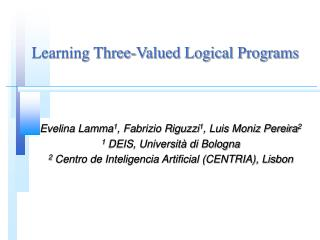DownloadDownload PresentationLearning Three-Valued Logical Programs

# Learning Three-Valued Logical Programs

Download Presentation## Learning Three-Valued Logical Programs

- - - - - - - - - - - - - - - - - - - - - - - - - - - E N D - - - - - - - - - - - - - - - - - - - - - - - - - - -
##### Presentation Transcript

1. Learning Three-Valued Logical Programs Evelina Lamma1, Fabrizio Riguzzi1, Luis Moniz Pereira2 1 DEIS, Università di Bologna 2 Centro de Inteligencia Artificial (CENTRIA), Lisbon

2. Extended Logic Programs • A finite set of rules of the form: L0 L1,...,Ln,,not Ln+1,...,not Lm where each Li can be either A or ¬A • ¬A is the explicit negation of A, ¬fliles(X)  animal(X). fliles(X)  bird(X). ¬ fliles(X)  penguin(X). • Three-valued semantics (WFSX)

3. Learning Three-Valued Logical Programs • Autonomous agent: acquisition of information by means of experiments • Experiment: • execution of an action • evaluation of the results with respect to the achievement of a goal • positive and negative results • Learning general rules on actions: • distinction among actions with a positive, negative or unknown outcome

4. New Learning Framework • Conditions on examples: P|E+, E-(completeness) P |E-, E+(consistency) • LIVE (Learning In a three-Valued Environment) • Standard ILP techniques to learn p and p • top-down or bottom-up • Learning of: • p using E+, E- as training set • p using E-, E+ as training set

5. Intersection of definitions  p p E+ E- Exceptions to the negative concept: positive examples Unseen atoms Exceptions to the positive concept: negative examples

6. Atoms in the intersection • Unseen atoms both true and false are classified as unknown: p(X)p+(X), notp(X). p(X)p-(X), not p(X). • Training set atoms must be classified according to the training set: p(X) p+(X), not abp(X), notp(X). p(X) p-(X), not abp(X), not p(X).

7. Generality of Solutions • Bottom-up methods: • search from specific to general: General Solution (GS) • GOLEM (RLGG), CIGOL (Inverse Resolution) • Top-down methods: • search from general to specific: Specific Solution (SS) • FOIL, Progol

8. Criteria for chosing the generality • Risk that can derive from a classification error • high risk GS • low risk SS • Confidence in the set of negative examples • high confidence GS • low confidence SS

9. E- flies+ E+ flies- d e a b c f Example B: bird(a). has_wings(a). jet(b). has_wings(b). angel(c). has_wings(c). has_limbs(c). penguin(d). has_wings(d). has_limbs(d). dog(e). has_limbs(e). cat(f). has_limbs(f). E+={flies(a)} E-={flies(d), flies(e)}

10. Strategies For Theory Refinement The theory can be revised differently depending on the type of literal that is found contradictory in the intersection.